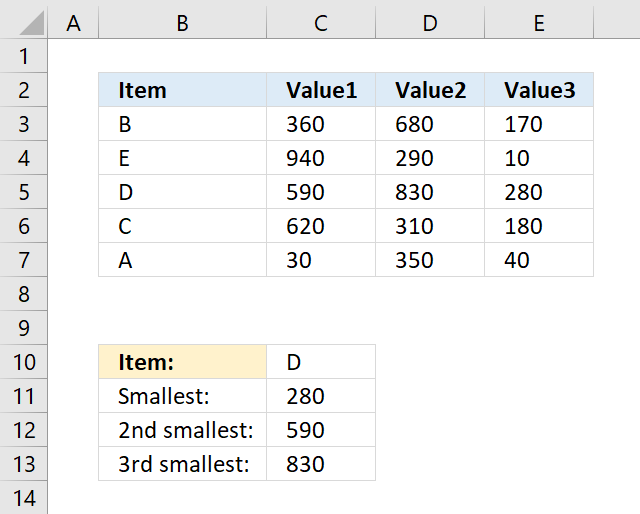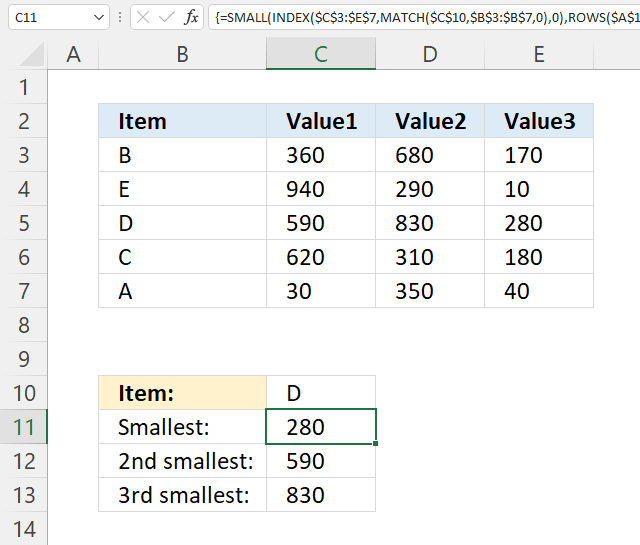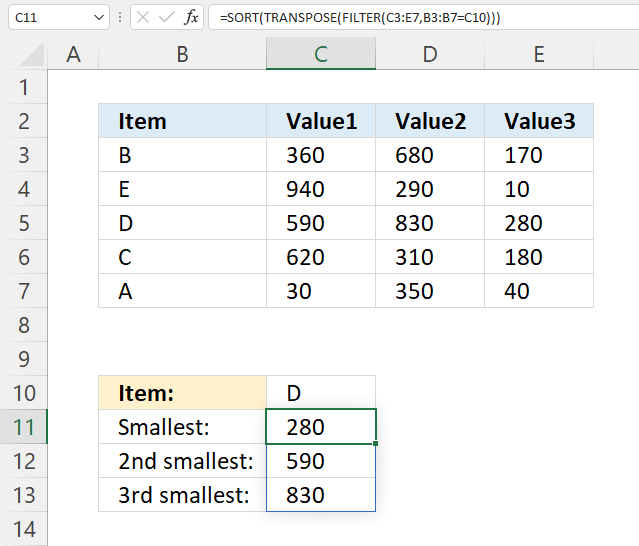Author: Oscar Cronquist Article last updated on July 09, 2022This article demonstrates how to extract multiple numbers based on a condition and return a sorted list from small to large.

The image above shows the formula in cell C11, it uses the specified value in cell C10 to determine which row it needs to extract numbers from. Cell B5 contains a value that is equal to the condition in cell C10.

The formula extracts the adjacent numbers on the same row and returns those numbers sorted from small to large in cell C11 and cells below as far as needed.

## 1. SMALL function - INDEXMATCHThe array formula in cell C11 gets 3 values in one fetch, the INDEX function allows you to do that if you enter 0 (zero) in the row or column argument. The SMALL function then calculates the k-th smallest value of these three values.

=SMALL(INDEX(\$C\$3:\$E\$7, MATCH(\$C\$10, \$B\$3:\$B\$7, 0), 0), ROWS(\$A\$1:A1))

### 1.1 How to enter an array formula

To enter an array formula, type the formula in a cell then press and hold CTRL + SHIFT simultaneously, now press Enter once. Release all keys.

The formula bar now shows the formula with a beginning and ending curly bracket telling you that you entered the formula successfully. Don't enter the curly brackets yourself.

### 1.2 Explaining formula in cell C11

#### Step 1 - Find the relative position of condition in B3:B7

The MATCH function returns the location of a specified value in an array och cell range.

MATCH(lookup_value, lookup_array, [match_type])

MATCH(\$C\$10, \$B\$3:\$B\$7, 0)

becomes

MATCH("D",{"B";"E";"D";"C";"A"},0)

and returns 3. Value D is found in position 3 in the array.

#### Step 2 - Get values based on relative position

The INDEX function returns a value from a cell range, you specify which value based on a row and column number. It can also return multiple values if you use 0 (zero) in the row or column argmunets or both.

INDEX(\$C\$3:\$E\$7, MATCH(\$C\$10, \$B\$3:\$B\$7, 0), 0)

becomes

INDEX(\$C\$3:\$E\$7, 3, 0)

The INDEX function then returns all values on relative row 3 in this cell range \$C\$3:\$E\$7: {590, 830, 280}

#### Step 3 - Extract k-th smallest number

The SMALL function returns the k-th smallest value from a group of numbers.

SMALL(array, k)

SMALL(INDEX(\$C\$3:\$E\$7, MATCH(\$C\$10, \$B\$3:\$B\$7, 0), 0), ROWS(\$A\$1:A1))

ROWS(\$A\$1:A1) returns the number of rows in cell reference \$A\$1:A1, it grows when you copy the cell and paste it to cells below. This makes the formula extract a new number in each cell below.

SMALL(INDEX(\$C\$3:\$E\$7, MATCH(\$C\$10, \$B\$3:\$B\$7, 0), 0), ROWS(\$A\$1:A1))

becomes

SMALL({590, 830, 280}, 1)

and returns 280 in cell C11.

You can also use the SMALL function to match multiple values, make sure you read this post: 5 easy ways to VLOOKUP and return multiple values

## 2. SMALL function - INDEXMATCH (Excel 365)The formula in cell C11 extracts numbers from a row that has a value that meets the condition. It then sorts the numbers from small to large.

Formula in cell C11:

=SORT(TRANSPOSE(FILTER(C3:E7, B3:B7=C10)))

### 2.1 Explaining formula in cell C11

#### Step 1 - Identify values that meet the condition

The equal sign lets you compare value to value, in this case, value to an array of values. The result is a boolean value TRUE or FALSE and the resulting array is equal in size to the array we compared.

B3:B7=C10

becomes

{"B"; "E"; "D"; "C"; "A"}="D"

and returns

{FALSE; FALSE; TRUE; FALSE; FALSE}.

#### Step 2 - Filter numbers

The FILTER function lets you extract values/rows based on a condition or criteria.

FILTER(arrayinclude, [if_empty])

FILTER(C3:E7, B3:B7=C10)

becomes

FILTER(C3:E7, {FALSE; FALSE; TRUE; FALSE; FALSE})

becomes

FILTER({360,680,170; 940,290,10; 590,830,280; 620,310,180; 30,350,40}, {FALSE; FALSE; TRUE; FALSE; FALSE})

and returns {590, 830, 280}.

Note that the numbers are comma-delimited meaning they are arranged horizontally.

#### Step 3 - Transpose values

The TRANSPOSE function allows you to convert a vertical range to a horizontal range, or vice versa.

TRANSPOSE(array)

TRANSPOSE(FILTER(C3:E7,B3:B7=C10))

becomes

TRANSPOSE({590, 830, 280})

and returns {590; 830; 280}.

#### Step 4 - Sort values

The SORT function lets you sort values from a cell range or array.

SORT(array, [sort_index], [sort_order], [by_col])

SORT(TRANSPOSE(FILTER(C3:E7,B3:B7=C10)))

becomes

SORT({590; 830; 280})

and returns {280; 590; 830}.